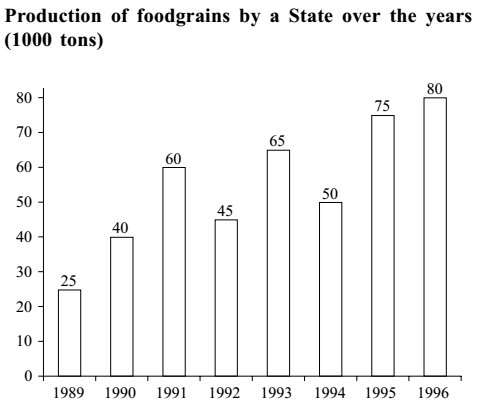#### Data Interpretation

Direction: Study the following graph carefully and answer the questions given below it.1. In which of the following years was the percentage increase in production from the previous year the maximum among the given years?
1. 1991
2. 1993
3. 1995
4. 1990
5. None of these
##### Correct Option: D

Check given option one by one.
Option (A),
Percentage increase in production in 1991 = {(Production in 1991 - Production in 1990)} x 100 % / Production in 1990
Percentage increase in production in 1991 = {( 60 - 40 )} x 100 % / 40
Percentage increase in production in 1991 = 20 x 100 % / 40
Percentage increase in production in 1991 = 100 % / 2 = 50 %
Option (B)
Percentage increase in production in 1993 = {( Production in 1993 - Production in 1992 )} x 100 % / Production in 1992
Percentage increase in production in 1993 = {( 65 - 45 )} x 100 % / 45
Percentage increase in production in 1993 = 20 x 100 % / 45
Percentage increase in production in 1993 = 4 x 100 % / 9 = 400/9 = 44.44 %
Option (C)
Percentage increase in production in 1995 = {( Production in 1995 - Production in 1994 )} x 100 % / Production in 1994
Percentage increase in production in 1995 = {( 75 - 50 )} x 100 % / 50
Percentage increase in production in 1995 = 25 x 100 % / 50
Percentage increase in production in 1995 = 100 % / 2 =50 %
Option (D)
Percentage increase in production in 1990 = {( Production in 1990 - Production in 1989 )} x 100 % / Production in 1989
Percentage increase in production in 1990 = {( 40 - 25)} x 100 % / 25
Percentage increase in production in 1990 = 15 x 100 % / 25
Percentage increase in production in 1990 = 15 x 4 % = 60 %